Example Questions

Example Question #74 : Probability

Aaron, Gary, Craig, and Boone are sitting down in a row of four chairs. What is the probability that Aaron and Gary will be seated beside each other?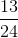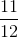Explanation:

Consider first all of the possible ways the men may be arranged, which is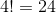Now, consider all of the ways that Aaron and Gary could be seated beside each other; it may be easier to visualize by drawing it out:

1. A G _ _
2. G A _ _
3. _ A G _
4. _ G A _
5. _ _ A G
6. _ _ G A

As seen, there are six possibilities.

Finally, for each of these cases, Craige and Boone could be seated in one of two ways.

So the probability that Aaron and Gary will be seated beside each other is: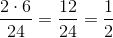Example Question #111 : How To Find The Probability Of An Outcome

Joaquin flips a coin t times.  It lands heads-up h times.  Which of the following represents the probability of the coin landing tails-up?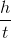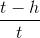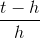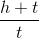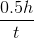Explanation:

A coin only has two outcomes, heads or tails.  So if the coin is flipped "t" times, "h" of those times are heads and "t-h" of those times are tails.

From here, we use the simple probability formula: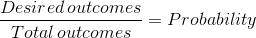sowe simply create a fraction that has the number of tails-up divied by the total number of flips;Example Question #112 : How To Find The Probability Of An Outcome

In Sarah's closet, there are 12 skirts. If she chooses one skirt at random, there is a 25% probability that the skirt is blue. How many blue skirts does Sarah have in her closet?Explanation:

The probability of any given event is given by the equation

P= [(number of desired events) / (total possible events)] x 100%

In this example, let x represent the number of blue skirts.

If there's a 25% probability that the skirt Sarah chooses is blue, then the probability of the event can be written like this:

P = (x/12)(100%) = 25%

To solve for x, we can set up a proportion like this: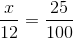By cross-multiplication, we get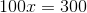Therefore, x=3.

Example Question #3191 : Sat Mathematics

Jennifer, James, and Kensington are flipping a coin and recording the outcomes for a math project about probability. What is the probability that the coin lands on tails, tails, tails, heads?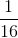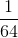Explanation:

In order to find the probability of the outcomes that Jennifer, James and Kensington flip, you need to multiply the probability of each outcome by each other.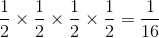Example Question #114 : How To Find The Probability Of An Outcome

There are seven scarves in a box. Three scarves are blue, two are red, and two are purple. Amelia picks one scarf out of the box to wear to school. What is the probability that the scarf she chooses at random is blue?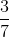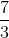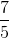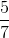Explanation:

The probability of the outcome of an event is given by the number of desired outcomes divided by the total number of possible outcomes.

For this example, since there are three blue scarves, there are three desired outcomes. In total, there are seven possible outcomes—one for each scarf Amelia could pick.

Therefore, the probability that she will pick a blue scarf isExample Question #115 : How To Find The Probability Of An Outcome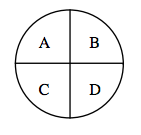On the spinner shown in the figure above, what is the probability of spinning an A, B, or C?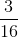Explanation:

The probability of spinning an A is. The probability of spinning an B is also, and the same goes for spinning a C.

To find the probability of spinning an A, B, or C, we simply add these individual probabilities together.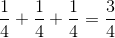Example Question #116 : How To Find The Probability Of An Outcome

Robert pulls the queen of hearts out of a deck of standard playing cards. He does not replace the card. Next, Rebecca pulls out a card of her own from the same deck. What is the probability that Rebecca's card is also a queen?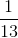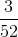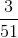Explanation:

Remember that we can find the probability of an event by dividing the number of possible desired outcomes by the total number of possible outcomes.

Since Robert has already pulled out the queen of hearts, that means that there are 51 cards remaining in the deck. That also means that there are only 3 queens left.

With this in mind, Rebecca pulls out a card from a deck of 51 total cards (the total number of possible outcomes), 3 of which are queens (the desired number of outcomes).

Therefore, the probability of her pulling out a queen isExample Question #117 : How To Find The Probability Of An Outcome

Joshua rolls a standard six-sided die and rolls a six. He rolls the same die a second time. What is the possibility that he rolls a six again?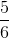Explanation:

The probability of an event is given by dividing the number of desired outcomes by the number of total outcomes.

Because each roll is an independent event, that means that the probability of rolling a second six is the same as rolling the first six.

The probability of rolling a six (one desired outcome) on a standard die (six total possible outcomes) isExample Question #281 : Data Analysis

There are two events,andIf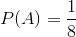and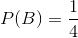, then what is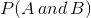?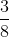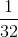Explanation:

Given two events, the following rule is true: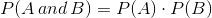Therefore,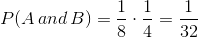Example Question #119 : How To Find The Probability Of An Outcome

In a jar, I have 5 red marbles, 6 blue marbles, and 4 green marbles. What is the probability that I will choose blue and red out of two picks?

In a jar, there have 5 red marbles, 6 blue marbles, and 4 green marbles. What is the probability that I will choose blue and red out of two picks?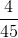Explanation:

Step 1: Find the probability of getting only one color. We will denote probability of red as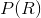, probability of blue as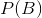and green as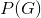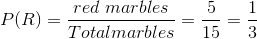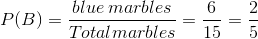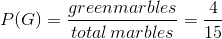.

This fraction cannot be simplified anymore. The numerator is divisible by 2 and the denominator is not divisible by 2.

Step 2: We need to find the probability of getting 1 red and 1 blue. We need to use a formula: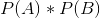, where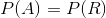and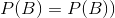.

Step 3: Using the formula in step 2, substituteandinto the equation and multiply.

We get: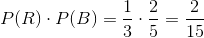So, the probability of getting a red and a blue marble is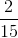.